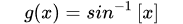Question Pool Inverse Trigonometric Functions

# Inverse Trigonometric Functions - SAMAGRA Question Pool & Answers | Class 12

Kerala Syllabus SAMAGRA SCERT SAMAGRA Question Pool for Class 12 Maths Inverse Trigonometric FunctionsQn 1.Get Free Study Materials + 1 Week Free Trial of BrainsPrep Class 12 Tuition

Qn 2.

(i) If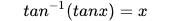, then which of the following is the value of x.

(a) 1.6 (b) 2 (c) -1.3 (d) -1.7

Consider the figure having 4 identical squares.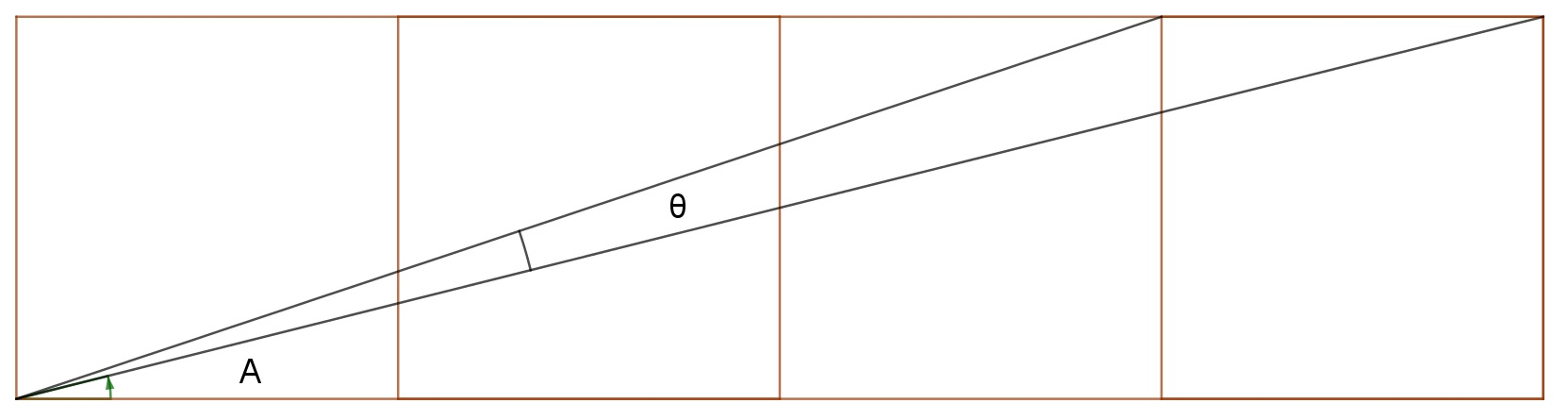(ii) Evaluate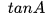(iii) Find the value of the angle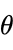Get Free Study Materials + 1 Week Free Trial of BrainsPrep Class 12 Tuition

Qn 3.

(i) Which of the following is true?(ii) Find x , if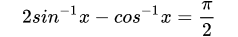Get Free Study Materials + 1 Week Free Trial of BrainsPrep Class 12 Tuition

Qn 4.

(i) Which of the following is the value of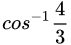(ii) If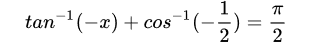,

then find the value of x.

Get Free Study Materials + 1 Week Free Trial of BrainsPrep Class 12 Tuition

Qn 5.

(i) If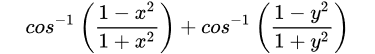,

where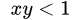, then show that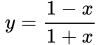(ii) Hence show that the function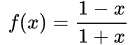is inverse of itself.

Get Free Study Materials + 1 Week Free Trial of BrainsPrep Class 12 Tuition

Qn 6.

(i) Which of the following can be the domain of the function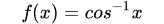(ii)If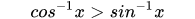, then find the interval in which x lies.

Get Free Study Materials + 1 Week Free Trial of BrainsPrep Class 12 Tuition

Qn 7.

(i) Which of the following is the value of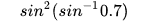?

(a) 0.7 (b) - 0.7 (c) 0.49 (d)0.14

(ii) Find the value of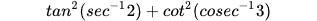Get Free Study Materials + 1 Week Free Trial of BrainsPrep Class 12 Tuition

Qn 8.

(i) Find the value of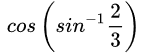(ii) If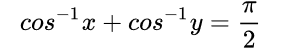, then show that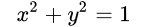(iii) Hence find x, if for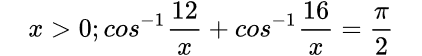Get Free Study Materials + 1 Week Free Trial of BrainsPrep Class 12 Tuition

Qn 9.

(i) Evaluate [-0.3],where [x] denotes the greastest integer function of x.

(ii) Which of the following is the domain of the function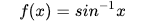?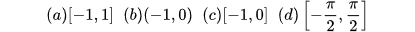(iii) Write the domain of the function